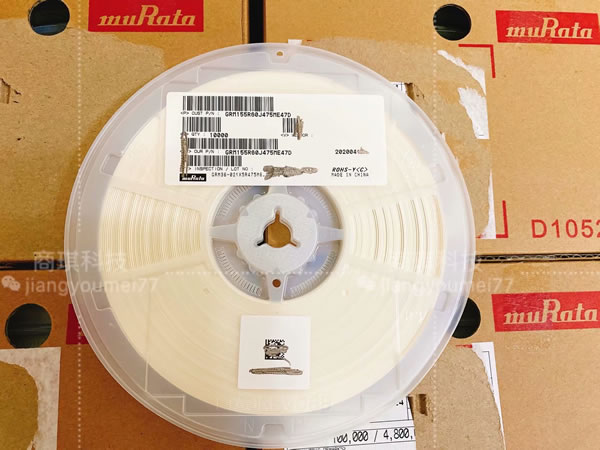• 全球服务热线：

0755-86009429
• 邮箱：

wendy@kcddz.com

US## 三星电容如何辨识选型-海金鑫

2021-03-29

CL03B104KQ8NNNC

1234567891011

1系列编码：

CL=积层陶瓷电容

2尺寸编码

03=0201(0603)21=0805(2012)42=1808(4520)05=0402(1005)31=1206(3216)43=1812(4532)10=0603(1608)32=1210(3225)55=2220(5750)14=0504(1410)01=0306(0816)12=0508(1220)

3介质：

I类II类

C=C0GS=S2HL=S2LP=P2HT=T2HR=R2HU=U2JA=X5RF=Y5VB=X7RX=X6S

4容量：

5电容的误差：

B=±0.1pfF=±1pf±1%K=±10%C=±0.25pfG=±2%M=±20%D=±0.5pf?J=±5%Z=+80/-20%

6额定电压：

R=4VO=16VB=50VE=250VI=1000VQ=6.3VA=25VC=100VG=500VJ=2000V

P=10VL=35VD=200VH=630VK=3000V

7厚度：

3=0.30毫米A=0.65毫米M=1.15毫米I=2.00毫米Q=1.25毫米5=0.50毫米C=0.85毫米F=1.25毫米J=2.50毫米V=2.50毫米8=0.80毫米D=1.00毫米H=1.60毫米L=3.20毫米

8内电极：

A=常规产品钯/银/镍屏蔽/锡100%；N=常规产品镍/铜/镍屏蔽/锡100%；G=常规产品铜/铜/镍屏蔽/锡100%：L=低侧面产品镍/铜/镍屏蔽/锡100%

9产品编码

A=阵列(2-元素)L=LICC；B=阵列(4-元素)N=常规；P=自动；C=高频

10特殊编码

11包装编码

B=散装O=纸版箱料带，10英寸料盘E=压花纸版箱，7英寸料盘；P=散装箱D=纸版箱料带，13英寸料盘(10000ea)F=压花纸版箱，13英寸料盘；C=纸版箱料带，7英寸料盘L=纸版箱料带，13英寸料盘(15，000ea)S=压花纸版箱，10英寸料盘。## 产品推荐

﻿